# Bypassing Pandas Memory Limitations

• Last Updated : 30 Apr, 2021

Pandas is a Python library used for analyzing and manipulating data sets but one of the major drawbacks of Pandas is memory limitation issues while working with large datasets since Pandas DataFrames (two-dimensional data structure) are kept in memory, there is a limit to how much data can be processed at a time.

Dataset in use: train_dataset

Attention geek! Strengthen your foundations with the Python Programming Foundation Course and learn the basics.

To begin with, your interview preparations Enhance your Data Structures concepts with the Python DS Course. And to begin with your Machine Learning Journey, join the Machine Learning - Basic Level Course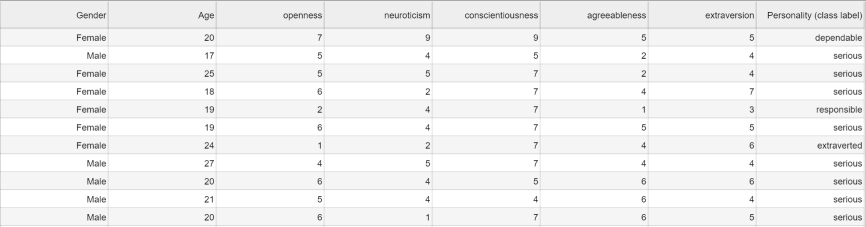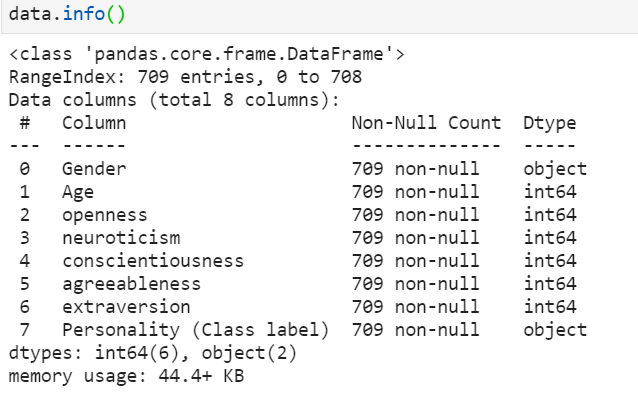Processing large amounts of data in Pandas requires one of the below approaches:

### Method 1: Load data in chunks

pandas.read_csv() has a parameter called chunksize which is used to load data in chunks. The parameter chunksize is the number of rows read at a time in a file by Pandas. It returns an iterator TextFileReader which needs to be iterated to get the data.

Syntax:

Example:

## Python3

 `import` `pandas as pd`` ` `data ``=` `pd.read_csv(``'train dataset.csv'``, chunksize``=``100``)`` ` `for` `x ``in` `data:``    ``print``(x.shape[``0``])`

Output:### Method 2: Filter out useful data

Large datasets have many columns/features but only some of them are actually used. So to save more time for data manipulation and computation, load only useful columns.

Syntax:

dataframe = dataframe[[‘column_1’, ‘column_2’, ‘column_3’, ‘column_4’, ‘column_5’]]

Example :

## Python3

 `import` `pandas as pd`` ` `data``=``pd.read_csv(``'train_dataset.csv'``)`` ` `data ``=` `data[[``'Gender'``, ``'Age'``, ``'openness'``, ``'neuroticism'``,``             ``'conscientiousness'``, ``'agreeableness'``, ``'extraversion'``]]`` ` `display(data)`

Output :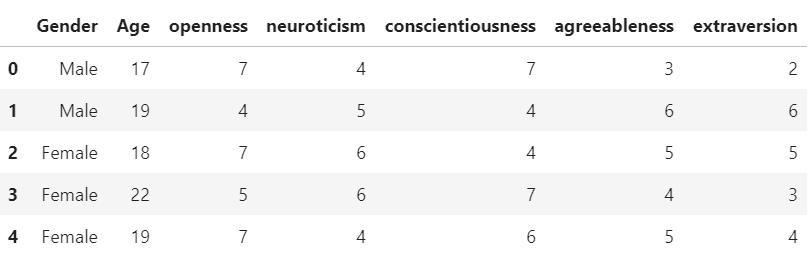### Method 3: Specify dtypes for columns

By default, pandas assigns int64 range(which is the largest available dtype) for all numeric values. But if the values in the numeric column are less than int64 range, then lesser capacity dtypes can be used to prevent extra memory allocation as larger dtypes use more memory.

Syntax:

Example :

## Python3

 `import` `pandas as pd`` ` `data ``=` `pd.read_csv(``'train_dataset.csv'``, dtype``=``{``'Age'``: ``'int32'``})`` ` `print``(data.info())`

Output :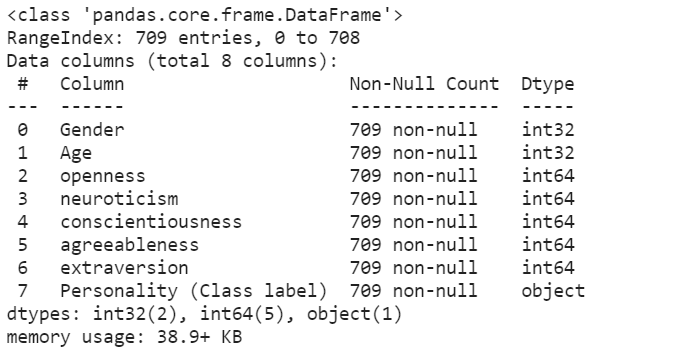### Method 4: Sparse data structures

Pandas Dataframe can be converted to Sparse Dataframe which means that any data matching a specific value is omitted in the representation. The sparse DataFrame allows for more efficient storage.

Syntax:

dataframe = dataFrame.to_sparse(fill_value=None, kind=’block’)

Since there are no null values in the above dataset, let’s create dataframe with some null values and convert it to a sparse dataframe.

Example :

## Python3

 `import` `pandas as pd``import` `numpy as np`` ` `df ``=` `pd.DataFrame(np.random.randn(``10000``, ``4``))``df.iloc[:``9998``] ``=` `np.nan`` ` `sdf ``=` `df.astype(pd.SparseDtype(``"float"``, np.nan))`` ` `sdf.head()`` ` `sdf.dtypes`

Output: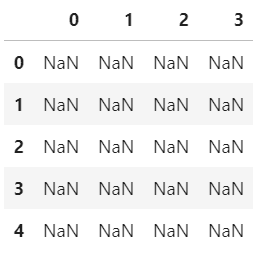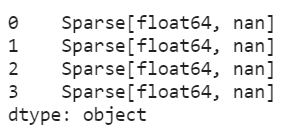### Method 5: Delete unused objects

While data cleaning/pre-processing many temporary data frames and objects are created which should be deleted after their use so that less memory is used. The del keyword in python is primarily used to delete objects in Python.

Syntax:

del object_name

Example :

## Python3

 `import` `pandas as pd`` ` `data ``=` `pd.read_csv(``'train_dataset.csv'``)`` ` `del` `data`

My Personal Notes arrow_drop_up